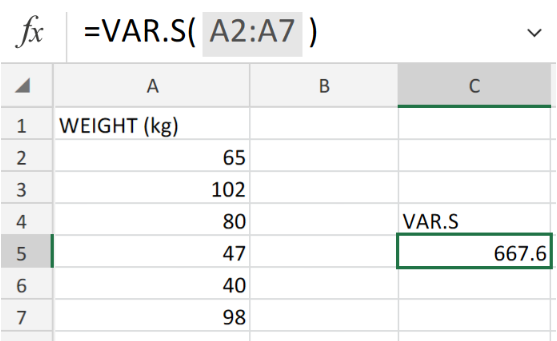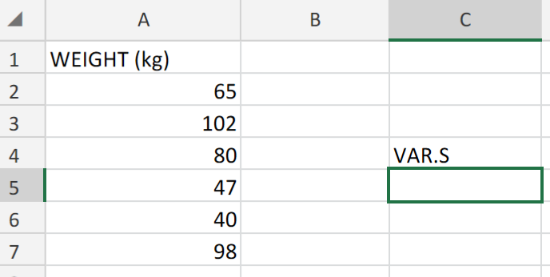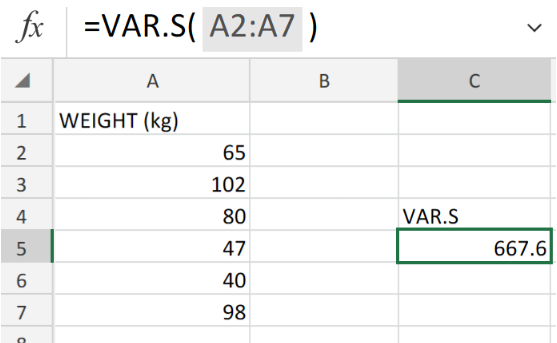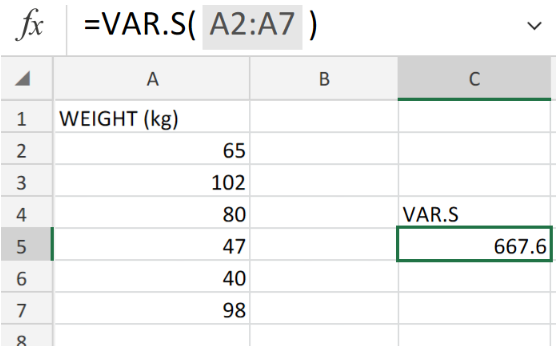Get instant live expert help with Excel or Google Sheets“My Excelchat expert helped me in less than 20 minutes, saving me what would have been 5 hours of work!”

#### Post your problem and you’ll get Expert help in seconds.

Your message must be at least 40 characters
Our professional Expert are available now. Your privacy is guaranteed.

# Excel VAR.S FunctionFigure 1. Excel VAR.S Function.

Let’s say we have a data set – which represents a whole population – and we want to determine its variance, we must use the Excel VAR.S Function.

## Generic Formula

`=VAR.S (number1, [number2], ...)`

• number 1 is the first reference or number.
• number 2 is the second reference or number (optional).

Our sample data variance can be determined by the VAR.S Function.

## How to use the Excel VAR.S Function.

Variance can provide us with an idea of the data spread across the entire population.

We are now going to make use of the Excel VAR.S Function to determine the variance of our data sample.

1. Arrange the data set in a column in our worksheet.Figure 2. Excel VAR.S Function.

Notice how we provided an empty cell for Excel to return the variance value.

1. In our worksheet example, the VAR.S formula to be entered into cell C5 is as follows;

`=VAR.S(A2:A7)`Figure 3. Excel VAR.S Function.

The calculated variance of the individual weight of the sample group, as shown in cell C5, is 667.6.

## Note

The Excel VAR.S function can accept up to 254 arguments/numbers.Figure 4. Final Result.

## Instant Connection to an Expert through our Excelchat Service:

Our live Excelchat Service is here for you. We have Excel Experts available 24/7 to answer any Excel questions you may have. Guaranteed connection within 30 seconds and a customized solution for you within 20 minutes.